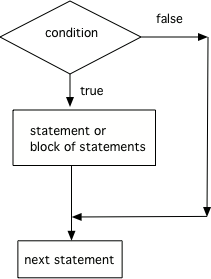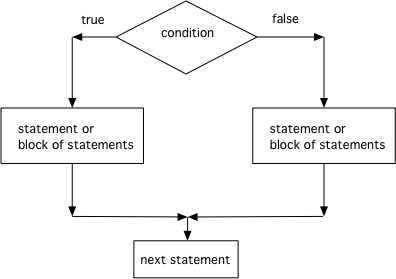5.1. Conditionals¶

Java statements normally execute one at a time from top to bottom. If you want a statement to only execute when something is true use a conditional. Something that can only be true or false is called a Boolean. If the condition is true then the next statement or a block of statements will execute. If the condition is false then the next statement or block of statements is skipped.Figure 1: The order that statements execute in a conditional

Note

A conditional uses the keyword `if` followed by Boolean expression inside of an open parenthesis `(` and a close parenthesis `)` and then followed by a single statement or block of statements. The single statement or block of statements are only executed if the condition is true. A block of statements is enclosed by an open curly brace `{` and a close curly brace `}`.

Imagine that your cell phone wanted to remind you to take an umbrella if it was currently raining in your area when it detected that you were leaving the house. This type of thing is going to become more common in the future and it is an area of research called Human Computer Interaction (HCI) or Ubiquitous Computing (computers are everywhere).

The variable `isRaining` is a boolean variable that is either true or false. If it is true then the message `Take an umbrella!` will be printed and then execution will continue with the next statement which will print `Drive carefully`. Run the code above to see this.

5-1-1: Try changing the code above to `boolean isRaining = false;`. What will it print?

What if you want to pick between two possibilities? If you are trying to decide between a couple of things to do, you might do one thing if a coin flip is heads and another if it is tails. In this case use the if keyword followed by a statement or block of statements and then the else keyword also followed by a statement or block of statements.Figure 2: The order that statements execute in a conditional with 2 options: if and else

Note

The else will only execute if the condition is false.

If `isHeads` is true it will print `Let's go to the game` and then `after conditional`. Run the code above to see this.

5-1-2: Try changing the code above to `boolean isHeads = false;`. What line will be printed before the `after conditional`?

Note

An if will only execute one single statement following it unless there is a block of statements enclosed in a pair of open and closed curly braces `{` and `}`. Java doesn’t care if you indent the code to show what you intend!

The code below doesn’t work as expected. Fix it to only print “Wear a coat” and “Wear gloves” when isCold is true.

5-1-3: What is the value of grade when the following code executes and score is 93?

```if (score >= 90) grade = "A";
if (score >= 80) grade = "B";
if (score >= 70) grade = "C";
if (score >= 60) grade = "D";
```
• A
• Notice that each of the first 4 statements start with an if. What will actually be printed? Try it.
• B
• Each of the first 4 if statements will execute.
• C
• Check this in DrJava.
• D
• Each of the if statements will be executed. So grade will be set to A, then B then C and finally D.
• E
• This will only be true when score is less than 60.

5-1-4: Which of the following is equivalent to the code segment below?

```if (x > 2) x = x * 2;
if (x > 4) x = 0;
```
• x = 0;
• If x was set to 1 then it would still equal 1.
• if (x > 2) x *= 2;
• What happens in the original when x is greater than 2?
• if (x > 2) x = 0;
• If x is greater than 2 it will be set to 0.
• if (x > 2) x = 0; else x *= 2;
• In the original what happens if x is less than 2? Does this give the same result?
Next Section - 5.2. Three or More Options